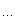## 条款2：尽量以CONST，ENUM，INLINE替换#DEFINE

const int GamePlayer::NumTurns;

enum{NumTurns = 5};

int scores[NumTurns];

posted @ 2009-12-21 00:18 蜜蜂 阅读(107) | 评论 (1)编辑 收藏

## vector

#include <vector>
#include
<string>
#include
<algorithm>
#include
<iterator>
#include
<iostream.h>
// #include <iostream>

class GreaterThan {
public:
GreaterThan(
int size = 6 ) : _size( size ){}

int size() { return _size; }

bool operator()(const string & s1) {

return s1.size() > 6;
}

private:

int _size;
};

template
<class Type>
class PrintElem {
public:

void operator()( const Type &elem )
{

++_cnt;

if ( _cnt % 8 == 0 ) { cout << endl; }
cout
<< elem << " ";
}

private:

static int _cnt;
};

template
< class Type >

int PrintElem<Type>::_cnt = 0;

class LessThan {
public:

bool operator()(const string & s1, const string & s2 ) {

return s1.size() < s2.size();
}
};

typedef vector
<string, allocator> textwords;

void process_vocab( vector<textwords, allocator> *pvec )
{

if ( ! pvec )

// issue warning message
return;

vector
< string, allocator > texts;

vector
<textwords, allocator>::iterator iter = pvec->begin();

for ( ; iter != pvec->end(); ++iter )
copy( (
*iter).begin(), (*iter).end(), back_inserter( texts ));

// sort the elements of texts
sort( texts.begin(), texts.end() );
for_each( texts.begin(), texts.end(), PrintElem
<string>() );

cout
<< endl << endl;

// delete all duplicate elements
vector<string, allocator>::iterator it;
it
= unique( texts.begin(), texts.end() );
texts.erase( it, texts.end() );
for_each( texts.begin(), texts.end(), PrintElem
<string>() );

cout
<< endl << endl;

stable_sort( texts.begin(), texts.end(), LessThan() );
for_each( texts.begin(), texts.end(), PrintElem
<string>() );

cout
<< endl << endl;

// count number of strings greater than length 6
int cnt = 0;

// obsolete form of count -- standard changes this
count_if( texts.begin(), texts.end(), GreaterThan(), cnt );

cout
<< "Number of words greater than length six are "

<< cnt << endl;

//static string rw[] = { "and""if""or""but""the" };
vector
<string,allocator> remove_words( rw, rw+5 );

vector
<string, allocator>::iterator it2 = remove_words.begin();

for ( ; it2 != remove_words.end(); ++it2 ) {

int cnt = 0;

// obsolete form of count -- standard changes this
count( texts.begin(), texts.end(), *it2, cnt );

cout
<< cnt << " instances removed:  "

<< (*it2) << endl;

texts.erase(
remove(texts.begin(),texts.end(),
*it2),
texts.end()
);
}

cout
<< endl << endl;
for_each( texts.begin(), texts.end(), PrintElem
<string>() );
}

typedef vector
<string,allocator>::difference_type diff_type;
#include
<fstream.h>

main()
{
vector
<textwords, allocator> sample;
vector
<string,allocator>       t1, t2;

string               t1fn, t2fn;

cout
<< "text file #1: "; cin >> t1fn;
cout
<< "text file #2: "; cin >> t2fn;

ifstream infile1( t1fn.c_str());
ifstream infile2( t2fn.c_str());

istream_iterator
< string, diff_type > input_set1( infile1 ), eos;
istream_iterator
< string, diff_type > input_set2( infile2 );

copy( input_set1, eos, back_inserter( t1 ));
copy( input_set2, eos, back_inserter( t2 ));

sample.push_back( t1 ); sample.push_back( t2 );
process_vocab(
&sample );
}

posted @ 2009-12-21 00:14 蜜蜂 阅读(117) | 评论 (0)编辑 收藏

 < 2020年10月 >
27282930123
45678910
11121314151617
18192021222324
25262728293031
1234567

• 随笔 - 2
• 文章 - 0
• 评论 - 1
• 引用 - 0

•